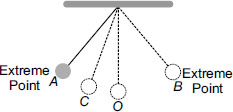# User Forum

Subject :NSO    Class : Class 8

A simple pendulum, oscillating with time period T, is shown in the figure. Time taken by the bob to move from A to C is t1 and from C to O is t2. ThenAMotion of the bob is uniform with T = 2(t1 + t2)
BMotion of the bob is uniform with T = 4(t1 + t2)
CMotion of the bob is non uniform with T = 2(t1 + t2)
DMotion of the bob is non-uniform with T = 4(t1 + t2).